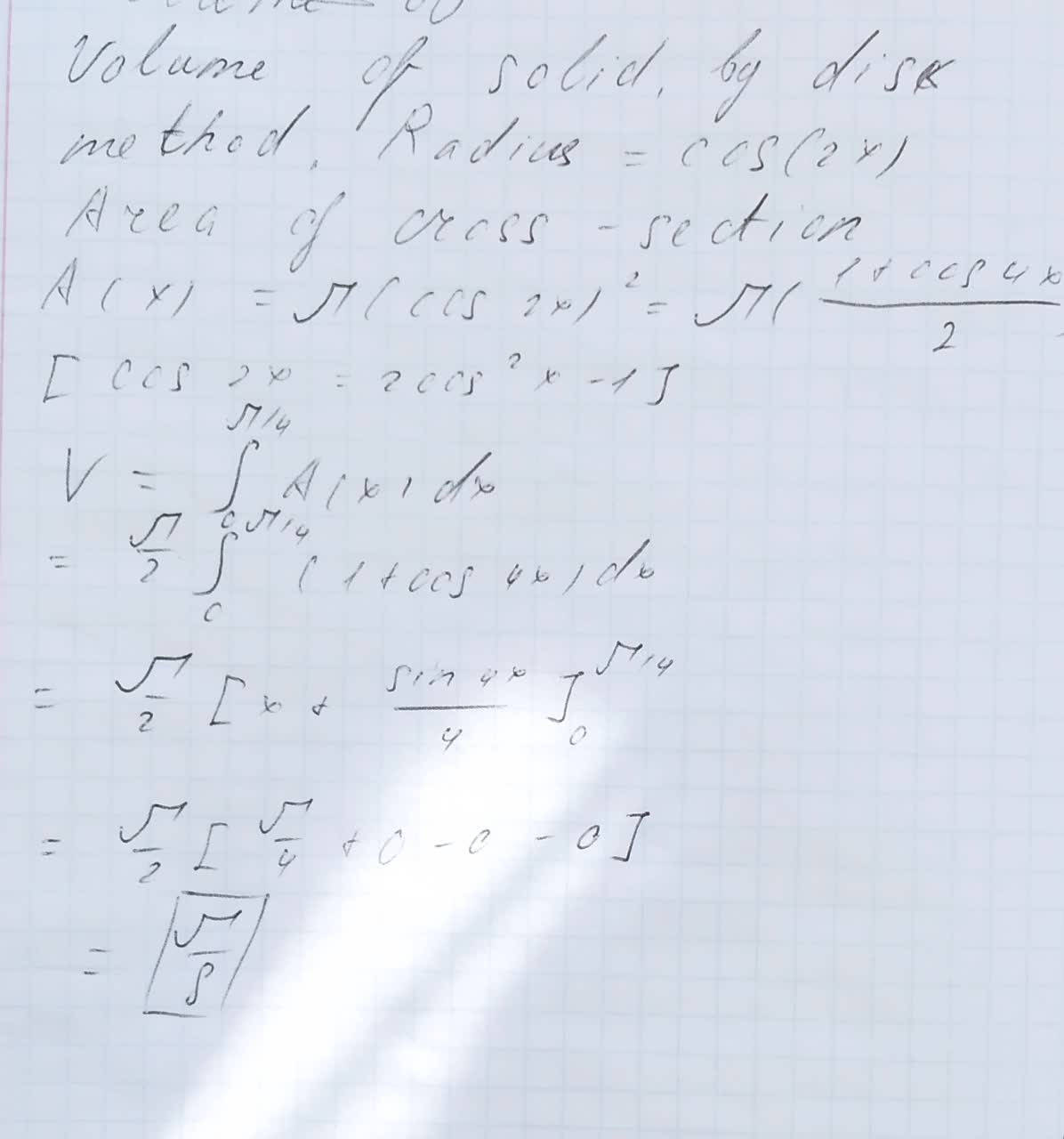# Find the volume of the solid generated by revolving the region bounded by the graphs of the equations about the x-axis. Verify your results using the integration capabilities of a graphing utility. y=cos 2x y=0 x=0 x=pi/4Tolnaio 2020-12-17 Answered
Find the volume of the solid generated by revolving the region bounded by the graphs of the equations about the x-axis. Verify your results using the integration capabilities of a graphing utility.
$y=\mathrm{cos}2x$
y=0
x=0
$x=\frac{\pi }{4}$
You can still ask an expert for help

• Questions are typically answered in as fast as 30 minutes

Solve your problem for the price of one coffee

• Math expert for every subject
• Pay only if we can solve itSchepperJ

Solved in photo###### Not exactly what you’re looking for?Jeffrey Jordon

Volume of solid by disk method. Radius $=\mathrm{cos}\left(2x\right)$

Area of cross section

$A\left(x\right)=\pi \left(\mathrm{cos}2x{\right)}^{2}=\pi \left(\frac{1+\mathrm{cos}4x}{2}\right)$

$\left[\mathrm{cos}2x=2{\mathrm{cos}}^{2}x-1\right]$

$V={\int }_{0}^{\pi /4}A\left(x\right)dx$

$=\frac{\pi }{2}{\int }_{0}^{\pi /4}\left(1+\mathrm{cos}4x\right)dx$

$=\frac{\pi }{2}\left[x+\frac{\mathrm{sin}4x}{4}{\right]}_{0}^{\pi /4}$

$=\frac{\pi }{2}\left[\frac{\pi }{4}+0-0-0\right]$

$=\frac{\pi }{8}$